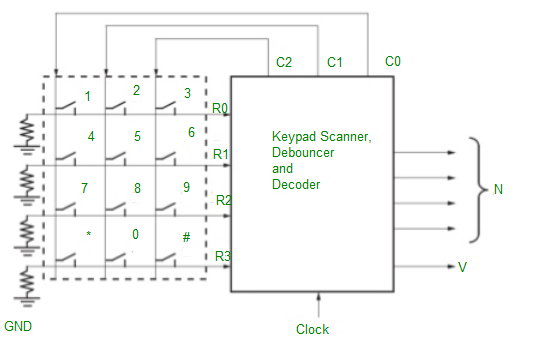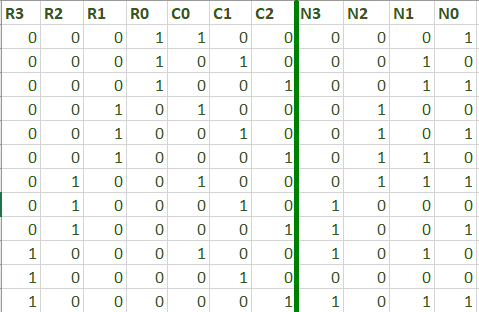A telephone keypad is designed using a PLD(Programmable Logic Device).
The keypad is wired in a matrix form with a switch at the intersection of each row and column. The purpose of this keypad scanner is to determine which has been pressed and output a binary number N=N3 N2 N1 N0 which corresponds to the key number. For example: Pressing the key ‘5’ will give the output 0101, Pressing ‘*’ key will give 1010 as the output.Whenever a valid key is detected, the scanner should output a signal V for on clock time T. Assuming one key is pressed at a time, resistors and ground are connected to each row of the keyboard so that R1=R2=R3=R4=0 when no key is pressed.

1. Apply logic 1 to columnsC0, C1, C2 and wait.
2. If any key is pressed, logic 1 will appear on corresponding resistor-R0, R1, R2 or R3.
3. Apply a logic 1 to column C0 only. If any of Resistors is 1, a valid key is detected. Set V=1 and the output is the corresponding N.
4. If no key is detected in the first column, apply a 1 to C1 and repeat. Repeat for C2 if no key is detected in the second column.
5. When a valid key is detected, apply 1s to C0, C1, C2 and wait until no key is pressed.
In the process of scanning a keyboard to determine which key is pressed, the scanner must take “Contact Bounce” into account. When the mechanical switch is opened or closed, the switch contact will bounce, causing noise to switch output.
6. Thus the output signal indicates that the key press must be synchronized with the clock.
Thus for a debouncing circuit, Clock Period > Bounce time.

The logic equations for the decoder are:

```N3 = R2(C0)' + R3(C1)'
N2 = R1 + R2CO
N1 = R0(C0)' + (R2)'C2 + (R1)'(R0)'(C0)
N0 = R1C1 + (R1)'C2 + (R3)'(R1)'(C1)'```

My Personal Notes arrow_drop_upCheck out this Author's contributed articles.

If you like GeeksforGeeks and would like to contribute, you can also write an article using contribute.geeksforgeeks.org or mail your article to contribute@geeksforgeeks.org. See your article appearing on the GeeksforGeeks main page and help other Geeks.

Please Improve this article if you find anything incorrect by clicking on the "Improve Article" button below.

Article Tags :

Be the First to upvote.

Please write to us at contribute@geeksforgeeks.org to report any issue with the above content.